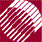ECE532 Biomedical Optics © 1998 Steven L. Jacques, Scott A. Prahl Oregon Graduate Institute

User settings

The user must select the settings that specify the simulation. Preset settings are listed for the following parameters:

• The optical properties of the medium:
• absorption coefficient (mua) = µa [cm-1]
• scattering coefficient (mus) = µs [cm-1]
• anisotropy (g) = g [dimensionless]
• refractive index (nt) = ntissue [dimensionless]
• The simulation parameters:
• number of photons to be launched (Nphotons).
• total range over which the bins extend (radial_size)
• number of bins for data output (NR). NR is preset to 100, and the array declarations declare the array sizes of Csph, Ccyl, and Cpla equal NR+1 = 101. If NR is changed, then the user must also change the array sizes declared in the variables section.

The albedo (albedo) and the incremental bin size (dr) are calculated for the user.

/**** INPUT
Input the optical properties
Input the bin and array sizes
Input the number of photons
*****/

mua         = 1.0;     /* cm^-1 */
mus         = 0.0;  /* cm^-1 */
g           = 0.90;
nt          = 1.33;
Nphotons    = 10000; /* set number of photons in simulation */
radial_size = 3.0;   /* cm, total range over which bins extend */
NR          = 100;	 /* set number of bins.  */
/* IF NR IS ALTERED, THEN USER MUST ALSO ALTER THE ARRAY DECLARATIONS */
/* for Csph, Ccyl, Cpla TO A SIZE = NR + 1. */
dr          = radial_size/NR;  /* cm */
albedo      = mus/(mus + mua);

to next page | Chapter 4 | Course | Home

©1998, Steven L. Jacques, Scott A. Prahl, Oregon Graduate Institute, Oregon Medical Laser Center